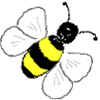#### You may also like### Speedy Sidney

Two trains set off at the same time from each end of a single straight railway line. A very fast bee starts off in front of the first train and flies continuously back and forth between the two trains. How far does Sidney fly before he is squashed between the two trains?### Temperature

Is there a temperature at which Celsius and Fahrenheit readings are the same?### Air Nets

Can you visualise whether these nets fold up into 3D shapes? Watch the videos each time to see if you were correct.

# Food Chains

##### Age 11 to 14Challenge Level

Well done to Amina from Greenacre Public School, Australia and Carl who sent us their solutions to this problem.

Amina found the ratio between the different organisms in the food chain and used it to find the answer to the first part or the problem:

a. The ratio is:
\begin{align} \begin{array}{ccccccc} \text{Bushes} &:& \text{Caterpillars}&:& \text{Birds}&:& \text{Wildcats}\\ 1 & :& 30 & :& 3 & :& 1 \\ 500 & :& 15000 & :& 1500 & :& 500 \end{array} \end{align}Therefore, if there are $500$ bushes, there will be $15000$ caterpillars, $1500$ birds and $500$ wildcats.

Carl sent us the following solutions to the other parts of the problem:

b. Each predator gets $100-0.25=0.75=75\%$ of the energy from the previous level of the food chain. So the caterpillars eat the bushes, and get $75\%$ of the energy. The birds eat the caterpillars and get $75\%$ of the caterpillars' energy, which is only $0.75^2=0.5625=56.25\%$ of the energy from the bush. So the wildcats get $75\%$ of the birds' energy, which is $0.75^3=0.421875=42.1875\%$ of the energy from the bush.

If the wildcats became vegetarian and ate the bush, they would get $75\%$ of the energy instead. So the wildcats could get $\frac{0.75-0.421875}{0.421875}=\frac{7}{9}=77.\dot{7}\%$ more energy by eating the bushes if they were vegetarian.

c. We still have $500$ bushes but now we only have $10000$ caterpillars, so we would then have:
$$\frac{10000}{10} = 1000 \text{ birds}\\ \frac{1000}{3} = 333 \text{ wildcats}$$This means that $500-333=167$ wildcats would starve due to a lack of food.

d. The wildcats only need to eat $56.25\%$ of a bush, so there is more food for the wildcats because a bush can feed $\frac{1}{0.5625} = \frac{16}{9} = 1.\dot{7}$ wildcats. So $500$ bushes would feed $500 \times \frac{16}{7} = 888$ wildcats.

e. In this (arguably fairer) situation $500$ bushes would feed $7500$ caterpillars and $444$ wildcats. This would allow for $750$ birds, which would then feed another $250$ wildcats. So in total there would be $500$ bushes, $7500$ caterpillars, $750$ birds and $694$ wildcats.